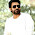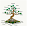» » Method and Type of methods

# Method and Type of methods

### Method:

• Method is a sub block of a class that contains logic of that class.
• logic must be placed inside a method, not directly at class level, if we place logic at class level compiler throws an error.
• So class level we are allowed to place variables and methods.
• The logical statements such as method calls, calculations and printing related statements must be placed inside method, because these statements are considered as logic.

1. package com.instanceofjava;
2. class sample{
3.
4. int a;
5. int b;
6.
7. System.out.println("instance of java"); // compiler throws an error.
8.
9. }
10.

1. package com.instanceofjava;
2. class sample{
3.
4. static int a=10;
5.
6. public static void main(String args[]){

7. System.out.println(a); // works fine,prints a value:10
8.
9.  }
10. }

### Method Terminology:

#### 1.Method Prototype:

• The head portion of the method is called method prototype.
1. package com.instanceofjava;
2. class sample{
3.
4. static int b=10;
5.
6. public static void main(String args[]) // ->method prototype.
7. {

8. System.out.println(b);
9.
10.  }

#### 2.Method body and logic:

• The "{ }" region is called method body, and the statements placed inside method body is called logic.

1. package com.instanceofjava;
2. class sample{

3. public static void main(String args[]) // ->method prototype.
4.
5.   int a=10,b=20; // method logic
6.   int c=a+b;  // method logic
7.   System.out.println(c);  // method logic
8.
9.  }
10. }

#### 3.Method parameters and arguments:

• The variables declared in method parenthesis "( )" are called parameters.
• We can define method with 0 to n number of parameters.
• The values passing to those parameters are called arguments.
• In method invocation we must pass arguments according to the parameters order and type.

1. package com.instanceofjava;
2. class sample{

3. public void add(int a, int b) // ->method prototype. int a, int b are parameters
4.
5.   int c=a+b;  // method logic
6.   System.out.println(c);  // method logic
7.
8.  }
9.
10. public static void main(String args[]) // ->method prototype.
11.
12.   sample obj= new sample();
13.
14.  obj.add(1,2); //method call , here 1, 2 are arguments.

15. }
16. }

#### 4:Method signature:

• The combination of method "name + parameters "  is called method signature

1. package com.instanceofjava;
2. class sample{

3. public void add(int a, int b) // ->method prototype. int a, int b are parameters
4.
5.   int c=a+b;  // method logic
6.   System.out.println(c);  // method logic
7.
8.  }
9.
10. public static void main(String args[]) // ->method prototype.
11.
12.   sample obj= new sample();
13.
14.  obj.add(1,2); //method call , here 1, 2 are arguments.

15. }
16. }

• In the above program add(int a, int b) and  main(String args[]) are method signatures.

#### 5. Method return type:

• The keyword that is placed before method name is called method return type.
• It tells to compiler and JVM about the type of the value is returned from this method after its execution
• If nothing is return by method then we can use "void" keyword which specifies method returns nothing.

1. package com.instanceofjava;
2. class sample{

3. public int add(int a, int b) // ->method prototype. int a, int b are parameters
4.
5.   int c=a+b;  // method logic
6.   return c;
7.  }
8.
9. public static void main(String args[]) // ->method prototype.
10.
11.   sample obj= new sample();
12.
13. int x= obj.add(1,2); //method call , here 1, 2 are arguments.
14. System.out.println(x);

15. }
16. }

#### 1.Method with out return type  and without arguments.

1. package com.instanceofjava;
2. class sample{
3.
4. public void add(){
5.
6. int a=10;
7. int b=10;
8. int c=a+b;
9. System.out.println(c);
10.
11. }

12. public static void main(String args[]) // ->method prototype.
13.
14. sample obj= new sample();
16.
17.
18.  }
19. }

#### 2.Method with out return type and with arguments.

1. package com.instanceofjava;
2. class sample{
3.
4. public void add(int a, int b){
5.
6. int c=a+b;
7. System.out.println(c);
8.
9. }

10. public static void main(String args[]) // ->method prototype.
11.
12. sample obj= new sample();
14.
15.
16.  }
17. }

#### 3.Method with return type  and without arguments.

1. package com.instanceofjava;
2. class sample{
3.
4. public int add(){
5. int a=20;
6. int b=30;
7. int c=a+b;
8. return c;
9. }

10. public static void main(String args[]) // ->method prototype.
11.
12. sample obj= new sample();
14. System.out.println(x);
15.
16.  }
17. }

#### 4.Method with return type and with arguments.

1. package com.instanceofjava;
2. class sample{
3.
4. public int add(int a, int b){
5.
6. int c=a+b;
7. return c;
8. }

9. public static void main(String args[]) // ->method prototype.
10.
11. sample obj= new sample();
12.
14. System.out.println(x);
15.
16.  }
17. }

#### Method terminology : main method

• Lets take main method and identify method parts

#### 1.Method Creation with body:

• The process of creating method with body is called method definition.
• Technically this method called concrete method.

1. class A{
2.
3. public void add(){
4.
5. }
6.
7. }

#### 2.Method Creation without body:

• Creating a method without body is called  "Declaring a method" or "Method declaration".
• Technically this method called "abstract method".
• In method declaration the modifier "abstract" is mandatory and also should be terminated with ";".
• Example : public void add();
• And only abstract class can have abstract method. (in interface we can use , by default all methods are abstract  )

1. abstract class A{
2.
3. abstract  public void add();
4.
5. }

### Static and non static methods:

• If a method has static keyword in its definition then that method called static method.
• We can call static method directly from main method.
• If method does not have static keyword in its definition then it is a non static method.
• If we want to call a non static method from main method we need object of that class
• if we want to call static method outside the class we can call by using classname.method();

1. class A{
2.
3. public static void show(){    // static method
4.  System.out.println("static method");
5.
6. public void display(){      //non static method
7.  System.out.println("non static method");
8.
9. public static void main(String[] args){
10. show(); // static method call
11. A obj= new A();
12. obj.display(); // non static method call
13. }
14. }

#### Instance Of Java

We will help you in learning.Please leave your comments and suggestions in comment section. if you any doubts please use search box provided right side. Search there for answers thank you.

#### 2 comments for Method and Type of methods

1.awesome explainations...)

2.Very good summary